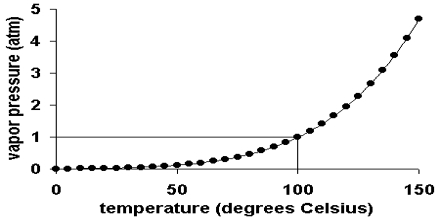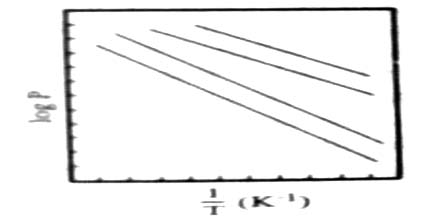Chemistry

# Vapour Pressure vs Temperature

Vapour Pressure vs Temperature

The general behavior of the vapour pressure of liquids at different temperatures is shown in Figure. It may be seen that vapour pressure rises rather sharply at higher temperatures. The curves continue to the critical temperature beyond which these cannot be extended because liquids cannot exist above the critical temperature.Fig: Vapour Pressure vs Temperature 1

As the temperature of a liquid rises, so does its vapor pressure. According to Kinetic Molecular Theory, the average energy of molecules increases as the temperature increases.

When logarithms of vapour pressure are plotted against the reciprocals of absolute temperatures straight lines, shown in Figure 2, and represented by the equation, where A and B are constraints.

log p = – B/T + A; are obtained.Fig: Log of vapor pressure vs 1/T

The slope B is equal to ∆Hvap/2.303 R where to ∆Hvap is the molar heat of vaporization. Hence the molar heat of vaporization may he determined from measurements of vapour pressure at several temperatures.

The vapour pressure of a pure substance at various temperatures is presented below.

T (°C) Pvap (mmHg)

• 10.0 → 20.0
• 15.0 → 36.3
• 25.0 → 52.2
• 40.0 → 86.3
• 60.0 → 157.2
• 80.0 → 267.5